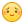Sclub交友聊天~加入聊天室當版主# [不等式] 两个最值

 已知$a,b,c$是非负实数，且$S=a+2b+3c,T=a+b^2+c^3$。 （1）求$T-S$的最小值； （2）若$S=4$，求$T$的最大值。

 （1）b^2+1>=2b，c^3+1+1>=3c；
 （2）由 S=4 知 b<=2, c<=4/3<√3，故 b^2<=2b, c^3<=3c，所以 T<=S=4，当 a=4,b=c=0 或 b=2,a=c=0 取等。 真无趣…………
 本帖最后由 isee 于 2021-10-14 22:06 编辑 回复 3# kuing     这是最近全国高中数学联合竞赛初赛B1加试第一题，哈哈哈哈哈哈
 回复 4# isee 纳尼？？？
 回复 5# kuing 试卷，自己看 https://www.gaokzx.com/c/202109/55976.html 摘 数学联赛：在每年9月中旬的第一个周日举行。目前联赛有一试、二试（加试）两场，同时还分为A卷和B卷。 联赛试题AB两套试卷主要是根据省份进行划分。浙江，江苏，河北，湖南，湖北，北京，上海，广东等绝大多数省份使用A卷；极少数偏远地区则使用B卷。B卷偏重对计算能力的考察，对思维方面的考察略低。由此可以看出北京赛区是使用A卷。
 回复 6# isee 好吧本帖最后由 lemondian 于 2021-10-15 14:24 编辑 修改一下： 问题（1）：已知$a,b,c$是非负实数，$a+2b+3c=4.$求$a+b^2+c^3$的最值。 能不能推广一下呢？ 问题（2）：已知$a_k\geqslant 0(k=1,2,\cdots ,n),\sum_{k=1}^nka_k=m$.求$\sum_{k=1}^na_k^k$的最值。
 显然乱改是没得玩嘀
 回复 9# kuing2021-10-15 16:40 我在网上看到的，但不会求
 回复 8# lemondian 问题（2）我是从这个题改的，不知能否推广（或在某些情况下推广）？ 已知$a,b,c$是非负实数，$a+b^2+c^3=9.$求$a+2b+3c$的最值。我在网上看到的，但不会求 lemondian 发表于 2021-10-15 16:41这个下界也没什么难度啊，消 a 变成： b,c>=0, 2b+3c<=4，求 f(b,c)=4-2b-3c+b^2+c^3 最小。 求导知 f(b,c) 的极值点是 (1,±1)，都不在可行域内，所以最小值一定在边界上。 于是只要分别求出 f(0,c), f(b,0), f((4-3c)/2,c) 的最小值即可。 易知前两个的分别为 2 和 3，第三个变成 (4-3c)^2/4+c^3 求导知 c=(\sqrt{41}-3)/4 时取最小值，结果就是图上那个，它比 2 小，所以最小值就是它。
 回复  lemondian 问题（2）我是从这个题改的，不知能否推广（或在某些情况下推广）？ 已知$a,b,c$是非负实数，$a+b^2+c^3=9.$求$a+2b+3c$的最值。 lemondian 发表于 2021-10-15 16:46最大值：$a+2b+3c\le a+b^2+1+c^3+1+1=12$，当 $a=7$, $b=c=1$ 取等； 最小值： 若 $c>2$，则 $a+2b+3c>6$； 若 $c\le2$，则 $3c\ge c^3$，显然 $b\le3$，故 $a+2b+3c\ge a+2b+c^3=9-b^2+2b=10-(b-1)^2\ge6$，当 $a=c=0$, $b=3$ 时取等。 综上，最小值为 6。 推广啥的你自己搞吧，我感觉没什么意思。
 回复 12# kuing 这个看不懂哩那就没办法了
 回复 12# kuing2021-10-17 23:54 终于来一个可以看得懂一些的了， 但好象还是有问题呀（图中红色部分处） 应该是$f(b,1)=1+(b-1)^2$ 另外，当$c=1$时，由$a+2b+3c=4$，可得$a+2b=1$，此时$b\in[0,1/2]$，所以$f(b,1)$的最小值应该是$5/4$呀。 @kuing
 回复 16# lemondian 沉了。。。
 返回列表 回复 发帖